Function Repository Resource:

# ModifiedAllanDeviation

Measure the bandwidth-modified two-sample phase/frequency stability

Contributed by: Julien Kluge
 ResourceFunction["ModifiedAllanDeviation"][data,r,taus] calculates the bandwidth-modified two-sample deviation of data along sample times taus at rate r.

## Details and Options

The modified Allan deviation is the square root of the bandwidth-modified two-sample variance, which is used to characterize the frequency/phase stability of an oscillator and can separate white from flicker noise.
The output usually comes in the format {τ,Around[σ,δσ]} where δσ denotes the statistical uncertainty related to the deviation σ.
The argument taus can be specified as:
 {{n}} attempts to place n logarithmically-spaced samples d places logarithmic spaced samplings with log base d {τ1,τ2,…} tries to use the specified sample times All uses all possible sample times Automatic tries to place a reasonable amount of samples along the whole possible time range
For a List of reals as a data argument, the resource function AllanDeviation will use a fast, compiled algorithm. Otherwise, valid inputs are exact expressions and/or Around.
The rate r must be a positive, numeric quantity.
Supported options are:
 "Overlapping" True whether the Allan deviation is overlapping "FrequencyData" False whether data is a list of frequencies or False for phase data

## Examples

### Basic Examples (2)

Calculate the overlapping modified Allan deviation of white noise at a rate of 0.1 for automatically chosen sampling points:

 In:=Out=Plot the results:

 In:=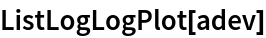Out=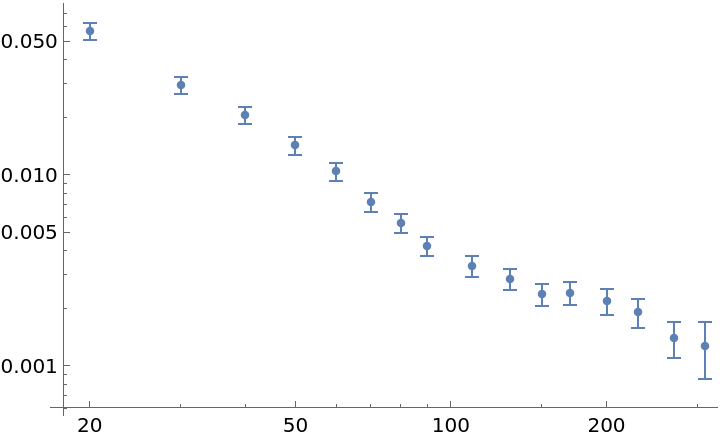Display the overlapping modified Allan deviation of white noise at a rate of 0.1 for certain sampling points:

 In:=Out=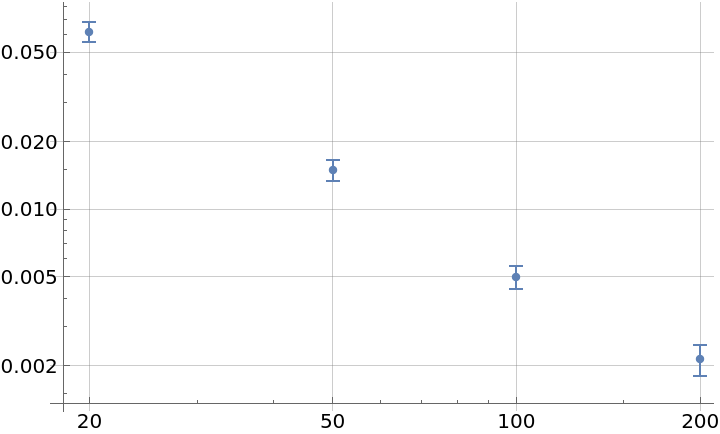### Scope (3)

Sample at all possible times:

 In:=Out=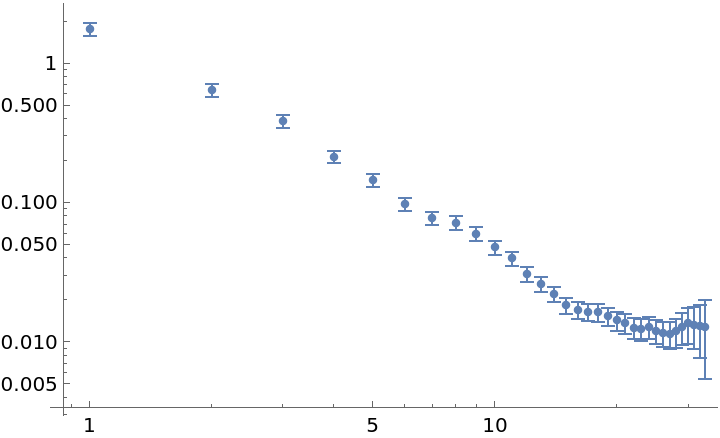Try to sample at five different time values:

 In:=Out=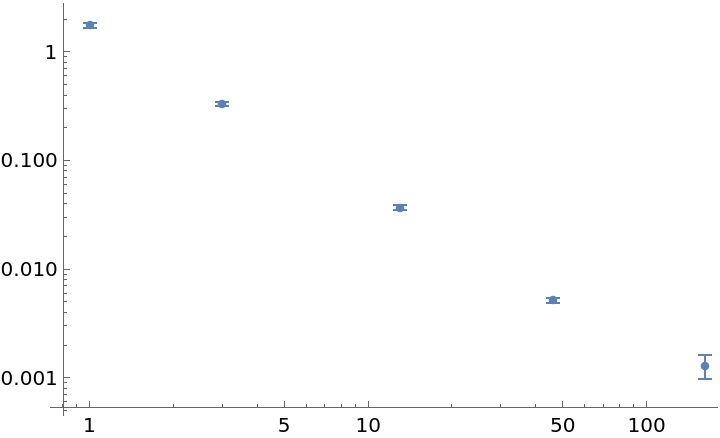The function will try to use a fast evaluation method when the data array is fully real, and otherwise prints a warning:

 In:=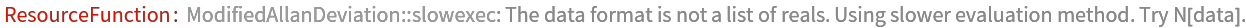Out=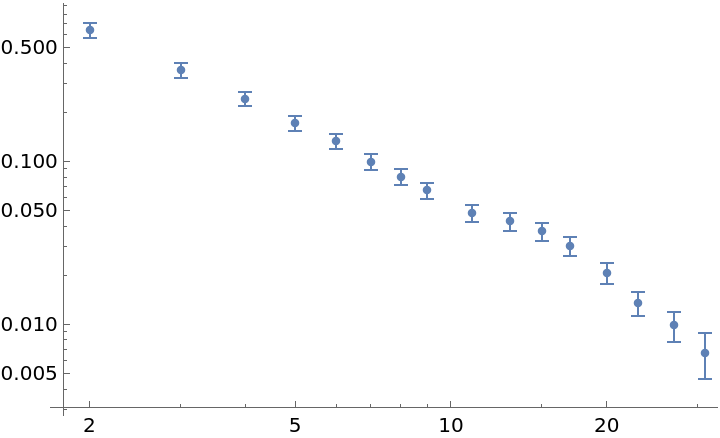### Options (2)

#### FrequencyData (1)

"FrequencyData" accepts a Boolean as argument. True signals the use of frequency data as opposed to phase data:

 In:=Out=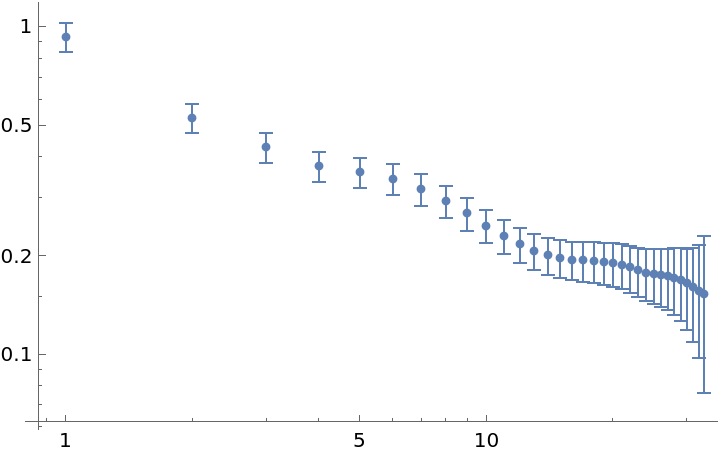#### Overlapping (1)

"Overlapping" accepts a Boolean as argument. False signals the use of non-overlapping strides in the deviation sampling:

 In:=Out=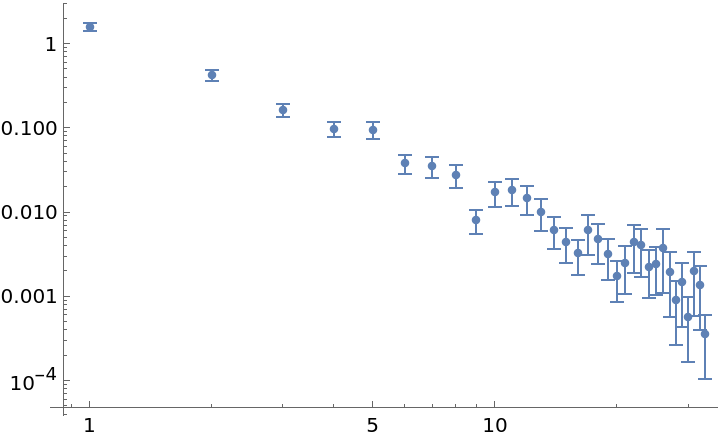### Applications (2)

Distinguish a random walk from white noise by their distinct slopes:

 In:=Out=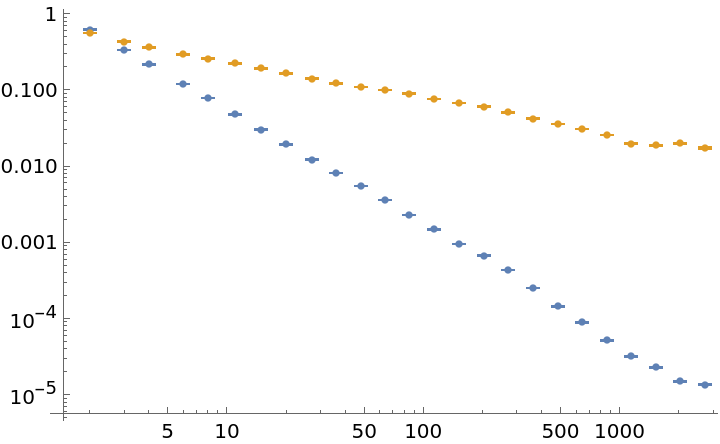Detect a drift in frequency data by a rising slope in longer sample times:

 In:=Out=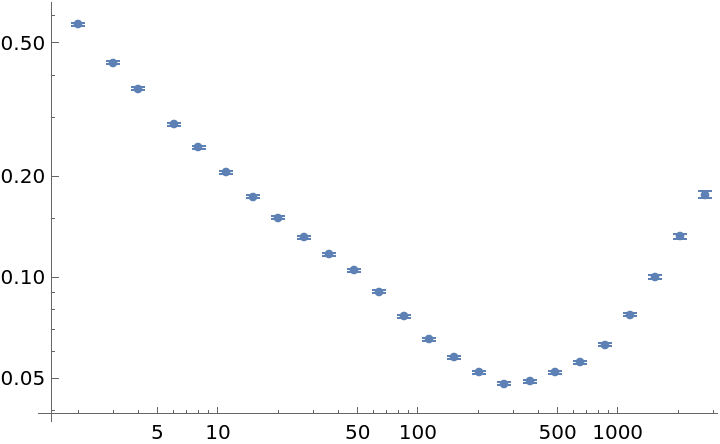### Properties and Relations (3)

Using the fast algorithm is approximately two times faster:

 In:=Out=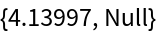In:=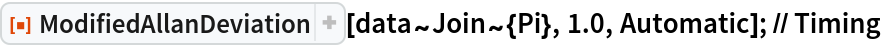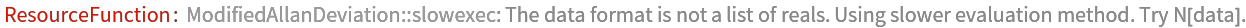Out=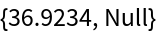The function can process Around objects as well as exact inputs directly:

 In:=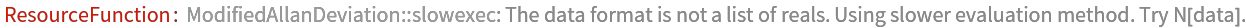Out=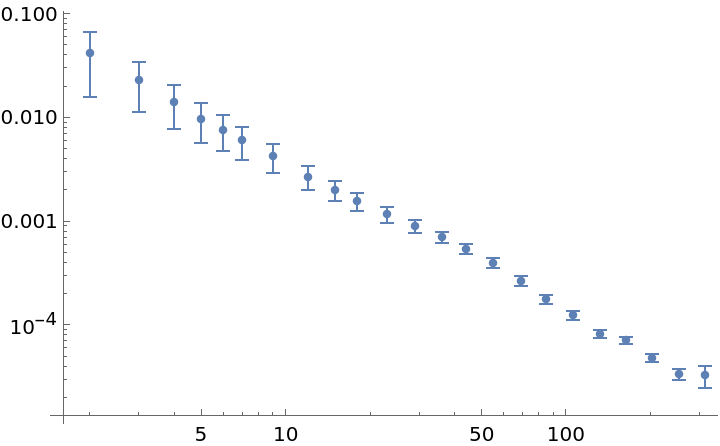Compare ModifiedAllanDeviation with the AllanDeviation resource function:

 In:=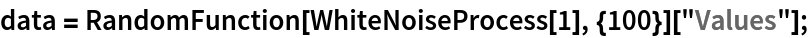In:=Out=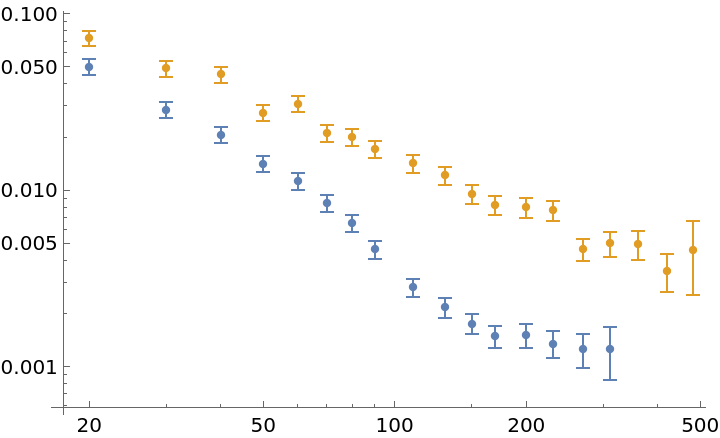### Possible Issues (1)

Requesting a certain amount of samples will not necessarily result in this amount of deviations:

 In:=Out=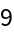### Neat Examples (1)

The function can distinguish between white phase noise and flicker noise (pink noise), as opposed to the plain Allan deviation:

 In:=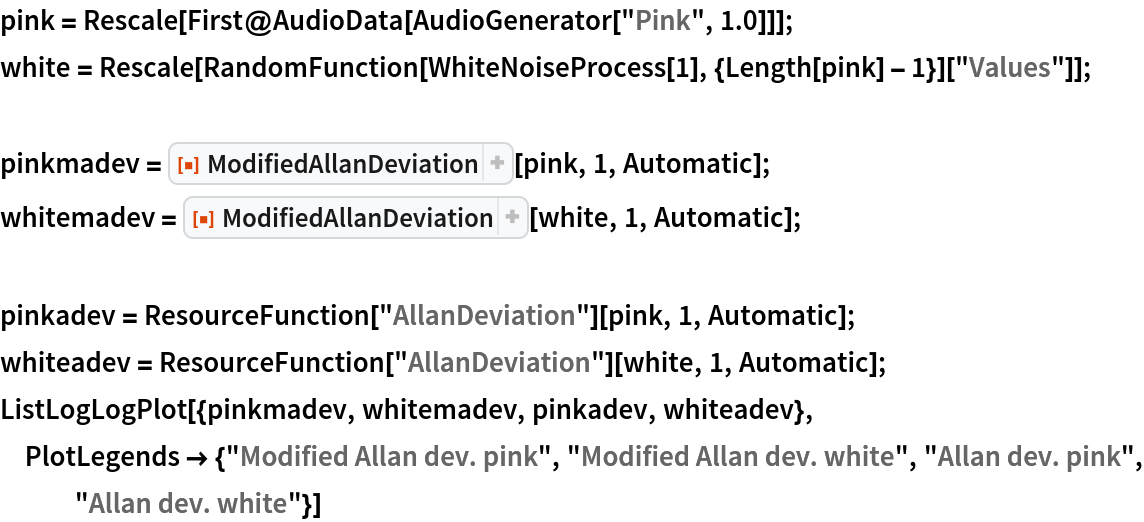Out=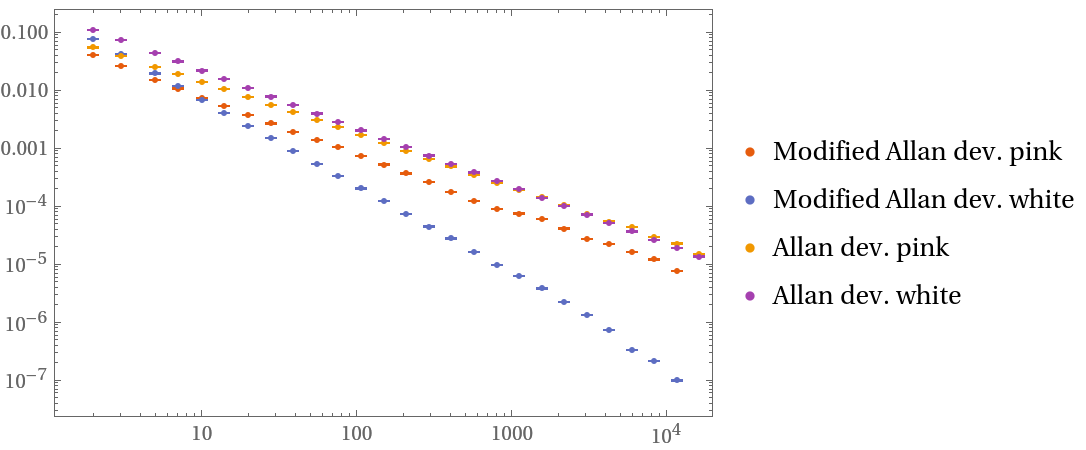Julien Kluge

## Version History

• 1.0.0 – 09 July 2020